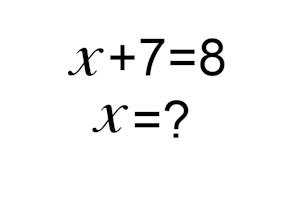• New Game

• Mute

• Game Mode

• HelpMaths Game - Basic Algebra

Maths Game - Basic Algebra was developed as an interactive tool to help you improve your maths skills by solving basic algebraic equations. The app is designed in the form of the simple game, with a scoring and level progression system. The objective of the game is to solve basic algebraic equations (i.e. find the value of an unknown variable - x). If you answer correctly you get a certain score. The difficulty of the game increase as you progress through the levels. Game mechanisms and the scoring structure is detailed in the help section.

Requirements: Internet browser with CSS3 support. Including: Internet Explorer 9+, Chrome 4+, Firefox 3.5+(partial support) 22+ (full support), Safari 4+, Opera 15+, iOS Safari 4.1+, Android Browser 2.3+, Chrome for Android 51+, IE Mobile 10+, UC Browser for Android 9.9+, Samsung Internet 4+. Older browsers only have partial functionality.

Android app Installs: Android 4.1+

Maths Game - Basic Algebra is provided free for personal use.

Version: 1.0Sinhala Alphabet - සිංහල හෝඩියSinhala Letters Writing PracticeMulti Language KeyboardHelaradio Sri Lanka Radio Stations appNomotion A Memory GameA Memory Game InmotionSinhala Transliteration ConverterSinhala Unicode ConverterEnglish to Sinhala TranslationSinhala to English TranslationSinhala Words ListSinhala Words - සිංහල වචනSinhala Words - Spelling GameSinhala KeyboardEnglish Alphabet Writing PracticeMaths Game - Elementary Arithmetic
• How to Play

The objective of the game is to solve basic algebraic equations that appears in the game screen (i.e. find the value of an unknown variable - x). Use the on screen keyboard to type your answer into the text box. The answer will be an absolute number. It can be a negative number. Use the ± (plus minus button) to insert/remove the negative sign. Then click the Ok button to see if you answered correctly. If you are correct a tick () appears. A cross () if answered incorrectly. You are given a score for a correct answer. The score is presented as partially filled or complete stars (see the Scoring section below). You can go to the following level by clicking the Next button. Here another algebraic problem appears and you need to repeat the process. Solving problems become more difficult as you progress through the levels. Initial equations only contain a single operator. In latter levels coefficients are included and multiple operations maybe required to solve the equation. In the Standard Mode there are 56 levels to complete the game. Quick and Long game modes have 28 and 112 levels respectively.

• Scoring

In the Maths Game - Basic Algebra app scores are presented as partially filled or complete stars. Scores are given for each correct answer. The weight of the score is based on the difficultly of the problem. At initial levels you are given a partially filled star for each correct answer, whereas, in the final levels you score a complete star for each correct answer. Cumulative tally of your star score is shown in the section above the game screen. The table below shows the weight of each scoring unit in the game.

• 5 X=• 5 X=• Game Complete Stats

The game is complete once you've attempted to answer the allotted number problems for the given game mode. Specifically, 28, 56 and 112 words for Quick, Standard and Long game mode respectively. Upon completion you'd be provided with several piece of information on your performance in the game. In the initial screen, along with your final score you will be given a mark for your performance. 'Perfect' performance means every equation was solved correctly. In the subsequent window detailed statistic will be displayed. Problems are rated and categorised according to difficulty. Number of correct and incorrect answers for each category is presented here.1x 1
+1=2
 x = 1?
 1x 1
+1=2
 x = 1?